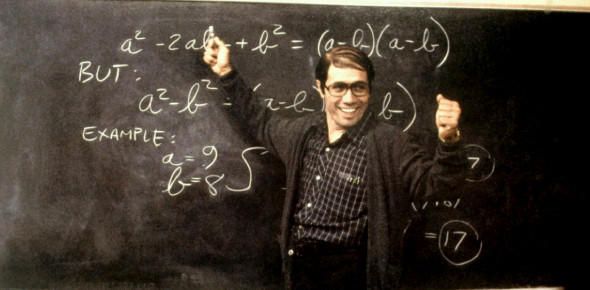# Test If You Are A Mathematics Genius!

8 Questions | Total Attempts: 619SettingsGenerally one requires to be a master in all but Jack in one. Our daily life directly or indirectly incorporates skills from the field of science and other subjects which may further embed the basic concepts of maths. Check if you fit in the boots of an average mathematician. This is so I can see what level you are in your math skill area. Go ahead. All the best!

• 1.
Why do you think math is important to learn in life.
• 2.
Janie had 24 cookies and wanted to fit all of them into four boxes. What is the correct soulution and the correct answer?
• A.

• B.

• C.

4x6=24= 6 cookies in each box

• 3.
Do you enjoy math?
• 4.
How do you define a circle?
• A.

It is a closed curve, which is drawn after taking a particular angle from a fixed point and then joining all the output points.

• B.

It is the locus of all points drawn from a perpendicular distance in a plane.

• C.

It is the locus of all the points drawn in a plane from a fixed point which is called as its centre.

• D.

None of the above

• 5.
Who discovered the concept of Zero?
• A.

Archimedes

• B.

Aryabhatta

• C.

Plato

• D.

None of the above

• 6.
The reciprocal of the secant value of an angle is equal to its cosecant.
• A.

True

• B.

False

• 7.
What is a null set?
• A.

A set which has zero as its element.

• B.

A set which is null.

• C.

A set which contains only one element.

• D.

None of the above.

• 8.
What is the value of the sum of squares of sin and cos of an angle?
• A.

1

• B.

2

• C.

0

• D.

-1

Related TopicsBack to top